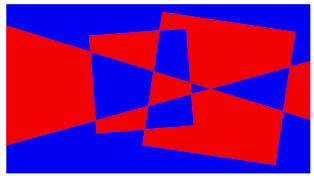# Two Colour Map TheoremAllPages RecentChanges Links to this page Edit this page Search Entry portal Advice For New Users

If a map is drawn such that each point on the boundary of a region is surrounded by an even number of regions then the map can be coloured in two colours.

That means that two colours are sufficient to colour such a map so that no two regions that share a common border are coloured with the same colour.

Consider any region, label it 0. Now consider any region bordering this region, label it 1. Continue labelling regions so that the label indicates the least number of steps required to pass from the region labelled 0 to that region.

All regions bordering a region labelled n will be labelled either n - 1 or n + 1. If two regions labelled 1 are neighbours then their corner point on the border with region labelled 0 will have an odd number of regions surrounding it. Similarly, if two regions labelled n are neighbours then their corner point on the boundary with the region labelled n - 1 will have an odd number of regions surounding it. Therefore two regions with the same label cannot be neighbours. So regions with an odd label can be coloured in one colour and regions with an even label can be coloured with the other.The theorem is equivalent to stating that all maps drawn with only infinite lines or closed loops are two colourable.

A proof by induction could be formed using this induction step:

• On a map in which the theorem is valid, draw a new line either an infinite line or closed loop.
• This line divides the region into two separate areas (inside/outside, top/bottom, left/right).
• The theorem is true in both areas however each and every region through which the line passes is divided into two where the theorem now fails.
• However flipping the colours of all the regions on one side of the new line (inside, top or left for example) will correct this fault whilst retaining the correctness of the theorem in both areas therefore generating a new and larger map in which the theorem is valid.
See Four Colour Theorem